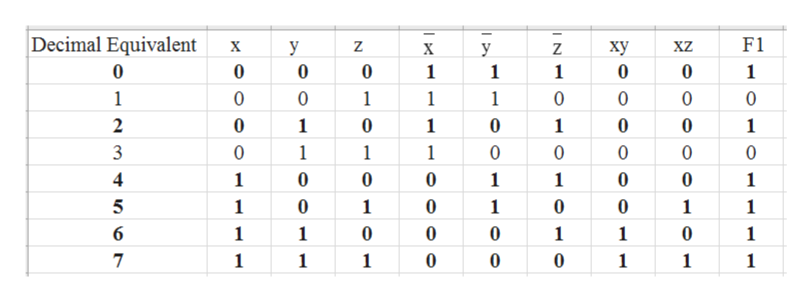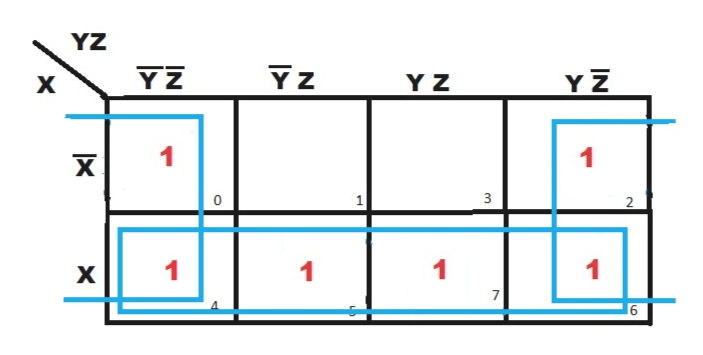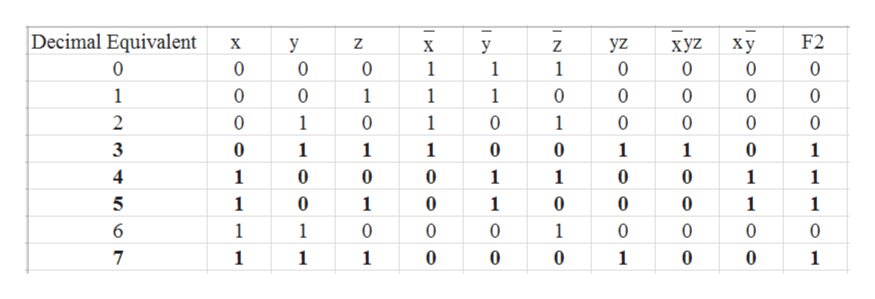PLEASE EXPLAIN ALL STEPS: This question has been answered multiple times, clearly by the same tutor, but I am seeing inconsistencies within the values, the explanation does not explain how these steps are accomplished, and I would very much appreciate another approach so that I do not continue to waste questions on explanations that are not providing any real understanding. Simplify the boolean equations using FIRST a truth table (one table for a, one table for b) and THEN forming a k-map (one k-map for a, one k-map for b):a. xz + (xy + ~z)b. ~xyz + yz + x~y

Question

PLEASE EXPLAIN ALL STEPS

This question has been answered multiple times, clearly by the same tutor, but I am seeing inconsistencies within the values, the explanation does not explain how these steps are accomplished, and I would very much appreciate another approach so that I do not continue to waste questions on explanations that are not providing any real understanding.

Simplify the boolean equations using FIRST a truth table (one table for a, one table for b) and THEN forming a k-map (one k-map for a, one k-map for b):

a. xz + (xy + ~z)

b. ~xyz + yz + x~y

Step 1

1)xz + (xy + ~z)

As there are three variables in the above Boolean expression there will be 23 = 8 cases in truth table.

The truth table is shown below for the given expression –

where F1= xz + (xy + ~z)help_outlineImage Transcriptionclose|Decimal Equivalent F1 X У Z ху XZ X y Z 0 0 0 0 0 0 1 1 1 1 1 1 0 0 1 1 0 0 2 1 0 1 0 0 1 1 0 1 1 1 0 0 0 0 0 4 1 0 0 1 0 1 1 1 0 1 0 1 1 1 6 0 0 1 0 1 1 1 1 0 7 1 0 0 1 1 1 1 1 fullscreen
Step 2

Now by using truth table, draw a K-map of 8 cells by following the steps given below-

a) Fill 1 at decimal equivalent number 0,4,2,5,6,7 as per the given question accordingly in the K-map.

b) Then first look for the octets, if there is no octet then search for quad and then for pairs and at the end if there is no such pair then search for singlet.

c)In this question it is clearly visible that there will be two quads formed in this K -map as shown below –

The final expression is shown below –

(x + ~z)help_outlineImage TranscriptioncloseYZ Yz Y Z Y Z Y Z х 1 1 3 2 1 1 1 1 7 6 X X fullscreen
Step 3

2) ~xyz + yz + x~y

As there are three variables in the above Boolean expression there will be 23 = 8 cases in truth table.

The truth ta...help_outlineImage TranscriptioncloseDecimal Equivalent F2 yz хуZ 0 х у Z ху 0 X y Z 0 0 0 0 1 1 0 0 1 1 0 0 1 1 1 0 0 0 0 0 1 1 2 0 1 0 0 0 0 0 3 0 1 1 1 1 1 1 0 0 0 0 1 1 1 1 1 5 0 0 0 0 1 1 1 1 1 6 1 0 0 1 0 0 0 1 0 7 0 0 1 1 1 1 1 fullscreen

Want to see the full answer?

See Solution

Want to see this answer and more?

Our solutions are written by experts, many with advanced degrees, and available 24/7

See Solution
Tagged in

Computer Science